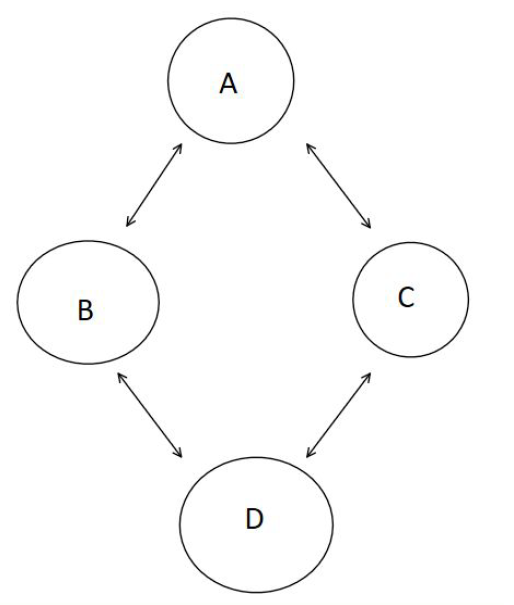# Graph Basics

### Introduction:

Graphs in computer science are different than what most people consider a graph to be. Graphs are a data type consisting of connected nodes (much like a linked list), only, with graphs, nodes have no limit to how many connections to other nodes they have.In a graph there is no head node, for example in the graph above, Node A is connected to Node B and C, B is connected to D, C is connected to A and D, and D is connected to C and B. As you can tell, there is no start to a graph, thus typically no head or first node.

Because of this, one must keep track of all the nodes in a graph, as each one can be a starting point for a specific path.

### Uses for Graphs:

Graphs are useful to store connections between data points and to show relationships among groups of data. For example, using a graph to display a group of friends on social media would be useful as the data (user’s) have unique connections that can be shown by the edges of a graph. Weighted graphs can be useful for things like real-time maps where there is a weight for the distance between two points (for example traffic).

### Types of Graphs:

Graphs can be either directed or undirected, depending on what their potential application is.

For an undirected graph, there is no direction of a connection, that is to say, a connection automatically goes both ways. For example, in a graph of Facebook users and their friends, there is no sense of direction between nodes (or users). If user A is friends with user B, then B is automatically friends with A. (You can’t be friends with someone without them being friends with you). The graph above is an undirected graph as there is no direction with respect to the connections, they can be crossed both ways.

For a directed graph, there is a sense of direction with each connection between nodes. For example in a graph of Twitter or Instagram user’s and their followers, just because A is following B, that does not mean B is following A. (You may follow a page or another individual but they do not automatically follow you back).

### Creating a Graph:

Graphs, like other data structures you have learned in this course, rely on using structs to store aspects of the graph. For the graphs you will be interacting with in this course, the graph will be a struct with every vertices stored within an array or a vector. These are stored in this manner because unlike a linked list, graphs have no official start value, so every vertices value must be stored as one can start from any point.

To create a graph, two things must occur: every vertex must be created and added to the graph (into the array/list/vector that stores the vertex) and every connection or edge between vertex must be created. In order to add edge between vertices, every vertice must already exist within the graph.

### Creating the Graph’s Edges:

For this class, the edges of a graph are stored within each vertex of the graph. Using a vector, stored within the struct vertex, each node or vertices stores information about the other vertices it is connected to. This means that given a node A, one can easily tell what other nodes it is connected to through the adjacent vertices vector. Because each edge is stored in the individual nodes, to create an edge, one must simply add the two nodes to each others adjacent vertices vector.

### Printing a Graph:

Because an array or vector of each vertice is stored within the graph, to print out each vertice and it’s connected nodes, one must loop through every vertice (through the array) and access each connected node to that vertice. Remember that all the information for the connected nodes to a vertex is stored within that vertex adjacent vertice matrix, which can also be looped through.

### Deleting From a Graph:

Because vertices in a graph do not have to be connected, to delete a vertex, one simply has to remove the vertex from the structure storing the vertices, and then remove it from every other vertex adjacency list, in essence, removing it from being a part of the graph and any path to traverse the graph.

### For Our Guides to Graph Traversing, Dijkstra’s Algorithm, and Heaps, Book a Session On Penji!## Penji Team

December 27th

View Profile

#### Get in Touch

Thanks! We'll reach out shortly.